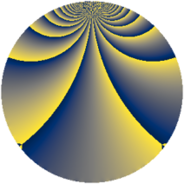# Properties

 Label 1110.2.beLevel $1110$ Weight $2$ Character orbit 1110.be Rep. character $\chi_{1110}(251,\cdot)$ Character field $\Q(\zeta_{12})$ Dimension $192$ Sturm bound $456$

# Related objects

## Defining parameters

 Level: $$N$$ $$=$$ $$1110 = 2 \cdot 3 \cdot 5 \cdot 37$$ Weight: $$k$$ $$=$$ $$2$$ Character orbit: $$[\chi]$$ $$=$$ 1110.be (of order $$12$$ and degree $$4$$) Character conductor: $$\operatorname{cond}(\chi)$$ $$=$$ $$111$$ Character field: $$\Q(\zeta_{12})$$ Sturm bound: $$456$$

## Dimensions

The following table gives the dimensions of various subspaces of $$M_{2}(1110, [\chi])$$.

Total New Old
Modular forms 944 192 752
Cusp forms 880 192 688
Eisenstein series 64 0 64

## Trace form

 $$192q + O(q^{10})$$ $$192q + 24q^{13} + 96q^{16} - 24q^{19} - 72q^{21} + 72q^{28} + 24q^{31} + 8q^{34} + 96q^{37} - 32q^{39} + 24q^{42} - 8q^{43} - 32q^{45} - 40q^{49} - 8q^{51} - 24q^{52} + 72q^{54} - 24q^{55} - 24q^{57} + 88q^{61} + 144q^{63} + 48q^{66} - 8q^{69} + 24q^{76} - 96q^{78} - 80q^{79} + 16q^{81} - 16q^{82} + 24q^{87} - 216q^{91} + 16q^{93} + 64q^{94} + 88q^{97} + 72q^{99} + O(q^{100})$$

## Decomposition of $$S_{2}^{\mathrm{new}}(1110, [\chi])$$ into newform subspaces

The newforms in this space have not yet been added to the LMFDB.

## Decomposition of $$S_{2}^{\mathrm{old}}(1110, [\chi])$$ into lower level spaces

$$S_{2}^{\mathrm{old}}(1110, [\chi]) \cong$$ $$S_{2}^{\mathrm{new}}(111, [\chi])$$$$^{\oplus 4}$$$$\oplus$$$$S_{2}^{\mathrm{new}}(222, [\chi])$$$$^{\oplus 2}$$$$\oplus$$$$S_{2}^{\mathrm{new}}(555, [\chi])$$$$^{\oplus 2}$$